# Week 3: Image Segmentation and Normalized Cuts

This week I will be looking at image segmentation and how it can be used to divide up an image into layers such that each segment can be properly upscaled individually.  This can be useful as it can allow for better edge retention after upscaling.

• Introduction
• Algorithm
• Implementation
• Results
• Final Discussion/Conclusion

Introduction

According to the paper, J. Shi and J. Malik proposed and analyzed the Normalized Cuts and Image Segmentation problem and trying to generate a general solution to this particular type of problems. This problem was brought up by Wertheimer around 85 years ago based on Graphic Theory, and is concerned with partitioning an image into multiple regions according to some homogeneity criterion.
As for this algorithm, it breaks down the targeted graph into a set of nodes, and each smallest unit of the graph, pixel, is regarded as the node of the graph. In addition, it regards the segmentation as graph partitioning problem. The algorithm measures both the total dissimilarity between the different groups as well as the total similarity within the groups. Thus, if we treat the problem in this manner, it will be transformed into a problem which requires solving the generalized eigenvalue.

Jianbo Shi and Jitendra Malik aim to extract global impressions of an image such as coherence of brightness, color and texture.  They approached the problem as a graph segmentation problem such that each segmented part will have one “global impression” attached to it, making all neighboring segmented parts different (bright vs dark segment).

In order to do this they had to consider existing algorithms used in graph theory to segment data based on minimizing certain parameters.  Such cuts are known as minimum cuts. These are cuts defined and computed based upon the minimum sum of weights of edges that have been removed.However as observed by Leahy and Wu, this method of partitioning based upon weightings on the edge cuts favors segmenting the graph into small sets of isolated nodes.  Contrary to this, Shi and Malik want to segment based on higher level traits which are “in general” larger segments. Thus they had to reformulate and define the cut equation to factor in these things.

The general idea behind the new formulation is that the decision to cut will be weighted as a cost based upon a node’s connections to other nodes.  This weighting will ensure that small section cuts will have a very high “normalized cut value” (recalling that small cut values are more desired) because we are cutting off a high percentage of all the nodes that were once connected to it.  Below is the definition of Ncut.

Defining the normalized association as follows will showCombining the two above equations we obtain.This shows that minimizing disassociation (minimizing Ncut) and maximizing association (maximizing Nassoc) are related and thus can be interchangeably used to to define cuts.
Although not discussed in this introduction the derivation and expansion of these algorithms will show an efficient way of segmenting images to produce separate images with individual properties.  Such as segmenting the individual sides of this cube.However, after the overall analysis, if we look back to this algorithm, the major issue would be the computation consumption.  In particular, the calculation of eigenvectors and weight matrix required a lot of time (using the traditional method O(n^3)), therefore, it makes this algorithm impractical when it comes to large images, and define the least possible node into a single pixel. Even though, efficiency can be dramatically improved by applying the normalized cut theorem (O(mn)) by solving it in a matrix-free fashion. However, as long as the resolution of the image goes higher, the computation is always ill-conditioned, which makes the complexity much higher.

When it comes to general applications, it would be easy for the program to overflow the buffer register provided by the low-end chipsets. As it requires a lot of construction sets, it would be impossible for them to perform in a real-time manner.
Nowadays, as the computation power goes higher, it enables the possibility for us to do it in a real time manner in higher level language and with higher level chipsets.

Algorithm

Knowing the concept of the normalized cut we can now understand the algorithm.  The algorithm is generalized as follows.

1. Given an image generate a graph G=(V,E) with vertices (V) and edges (E) and calculate the weights on each edge (w).
2. Solve the equation(discussed below) for the second smallest eigenvalue and eigenvector
3. Use the second smallest eigenvalue and eigenvector to calculate the minimum normalized cut and partition the graph into two new graphs
4. Determine whether the two new graphs can be partitioned again given a minimum Ncut condition (which we set)
5. If step four is valid we will recursively call the algorithm to partition again.

In order to calculate all the normalized cuts necessary we will need to solve the following equation.In this equation there are several variables to define.

1.: This is defined as an N=|V| dimensional indicator to mark whether a point is in segment A (1) or segment B (-1)
2.: This is the final calculated N cut for the input of x.
3.: Where w represents the weight of a node at point (i,j)
4.: N by N diagonal matrix with the weights d(i) on the diagonal
5.: N by N symmetric matrix with W(i,j)=w_ij
6.:7.8.:9.: Transpose of y

Solving the above for real values of y means we need to solve the following eigenvalue system.By solving this we obtain two important things, the eigenvalues, and eigenvectors of the system.  Because of the Rayleigh quotient we know that given a symmetric matrix the minimum value of an eigenvalue matrix is the second eigenvalue.

Going through the computation and linear algebra we obtain:The second smallest eigenvalue. Where z is defined as.Implementation
The implementation of the code in Matlab will have to be split into several parts.  The flowchart of the program has been shown below.  The full implementation of the code will be attached to this paper.In the calculation of the weights the Gaussian function is used.  Shi and Malik found that this function (given selected scaling factors) is a good representation of the weight on each edge.  The reason for choosing this is that the function is really good for representing singularities such as edges and sharp changes in contrast.  In this implementation the weight represents the difference in brightness between two pixels.  The closer the difference between the brightness, the more likely these two pixels are part of the same segment.  The larger the difference between the brightness of two pixels, the less likely they are part of the same segment  This results in the following equation for calculating the weights.

Here the variables in the equation are defined as follows

1. F is defined as a feature vector representing brightness (can be intensity, color, or texture information)
2.andis a scaling vector that Shi and Malik found should be set to 10-20% of the range of max(d)-min(d)
3.represents the euclidean distance between F(i) and F(j)
4. X represents the spatial location of the nodeResults

The implementation in Matlab is incredibly inefficient in its current state.  After investigating  Shi and Malik’s C implementation of the algorithm, we believe this is due to incredibly memory intensive computation in Ncuts.m.  There should be a way to circumvent the 8-9 gigabyte ram requirement by setting a second condition when checking whether we have reached the end of our recursion.  Namely, we need to set a minimum area.  This is more efficient than performing an Ncut computation and thus will be much faster to implement.  However, we are not sure what area size to pick and as such the an exact solution escapes us.  Below is an example of the algorithm with a run time of ~2minutes.Final Discussion/Conclusion

This algorithm developed by Shi and Malik is simple and ingenious.  It takes a traditional concept of the graph cuts and extends it so that instead of mathematically optimizing cuts, we take normalized cuts which depending on the feature vector used can be adapted to calculate cuts based on perceptual grouping through their idea of normalized cuts.  By solving a generalized eigenvalue system for a real valued solution, previously deemed impossible.

In our implementation of the algorithm though inefficient, follows logically from the description and original C implementation provided by Shi and Malik themselves.  This can be easily seen as Shi and Malik’s algorithm has been proved to be O(mn), contrasted to ours of unknown but (definitely) slower implementation.  This can definitely be improved by improving the algorithm and change of hardware as Shi and Malik’s algorithm uses a lot of matrix operations which can easily be parallelized.

In conclusion, we believe after thoroughly reading the paper and implementing a rudimentary version of the algorithm we have gained a strong understanding of image segmentation and how it can be used to segment images based on parameters relating to human perception.

Sources:

Shi, Jianbo, and Jitendra Malik. “Normalized Cuts and Image Segmentation.” IEEE TRANSACTIONS ON PATTERN ANALYSIS AND MACHINE INTELLIGENCE 22.8 (2000): 888-905. Web.

http://pastebin.com/cQjc0Php

# Week 2: Image and Video Upscaling from Local Self-Examples

Definitions

• Dyadic Filters: Filters that implement that use octave bands.
• Octave band filters: These filters separate the complete frequency spectrum into a set of frequencies.
• Octave band: An octave band is defined when upper band frequency is twice the lower band frequency.
• 1/3 Octave band filter: Defined when upper band frequency is the lower and freq times cube root of two ($f_{high}=f_{low}\sqrt{2}$)

This post is based on the paper written by GILAD FREEDMAN and RAANAN FATTAL at the Hebrew University of Jerusalem.  I will be trying to analyze and provide an overview and hopefully provide a complete understanding about how their image upscaling algorithm works.

Concept

Freedman and Fattal’s upscaling algorithm is based on the idea that in a certain group of pixels, other nearby pixels will present a similarity.  Using this idea, their algorithm will be able gather example data and produce an educated interpolation based upon this.  Hence the title of the paper “Local Self Examples”.  They contrasted their algorithm with global searches and database searches and showed that their algorithm is almost an order faster than many others because there is no need to expand the search to a significantly larger search radius.

It is relevant to note that due to the small size of the search area, using large upscaling factors will be detrimental and produce undesired results.  In order to scale to a larger factor one must run the algorithm multiple times.

In addition to this they are using the YCbCr color scheme as it has proven to be faster than the RGB color scheme.  This is because they only had to modify the Y component instead of all three of the RGB components.

Local Self-Similarity

The idea here is that the source image has the most similarities in it because that is the picture you’re trying to process.  Thus compared to external databases with high resolution images (but not your exact image) it is easy to see why similarities are much more prevalent.

That begs the question what similarities are we using to predict for interpolation?  One of the largest problems with image upscaling is retaining the sharpness of edges in images .SourceUpscaled using vectorization

Thus it would only be natural to use these edges of the source image as patches of “similarity”.  In addition because they come from the same source image, Freedman and Fattal call this local self-similarity.

However, these patches are only several pixels wide.  Quoting their experimental results, using patches of 5×5 pixels with search area 10×10 pixels (Local) and 20×20 pixels (Local 2) they show that on scale with error versus time required to process (noting small scaling factor of 5(upscaled):4(source)) that their algorithm is much superior.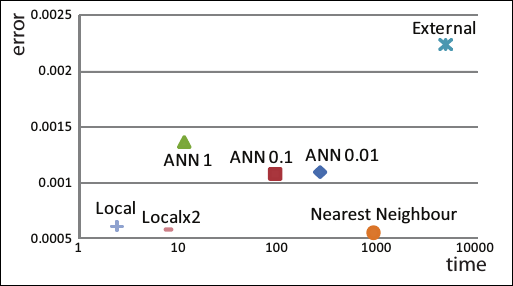Fig 9 from Freedman and Fattal: External=External database sources, Nearest Neighbor: linear interpolation, ANN: Approximate Nearest Neighbor: linear interpolation

Algorithm

The algorithm can be defined in the following steps. Given input image$I_{0}$. Operators for upsampling and downsampling are given using the non-dyadic filters design by Freedman and Fattal.

1. Use interpolation factor U to create a finer grid of pixels from grid$G_{l}$ to$G_{l+1}$
•$L_{1}=U(I_{0})$
2.  Because$L_{1}$ is lacking high frequency components, due to attenuation, of the source image obtain high frequency components from the source image to produce$L_{0}$ (low freq content smoothed image) where D is defined as the downsampling operator which maps$G_{l}$ to$G_{l-1}$
•$L_{0}=U(D(I_{0}))$
3. Compare local patches$p$ (small radius) of$G_{1}$ to$G_{0}$ the most similar patch in$G_{0}$ defined as$q$
4. Compute the lacking high frequency content in$L_{1}$ by doing the following, where$q$ represent the low frequency locations in the source image
•$H_{0}=I_{0}(q)-L_{0}(q)$
5. Add the high frequency image to the interpolated$L_{1}$ and compute final output image
•$I_{p}=L_{1}(p)+H_{0}(q(p))$

Conditions for using Freedman and Fattal’s algorithm

1. Uniform Scaling: Every pixel mapped from an upscale or downsample to a new image must be the same distance from each other
•$L_{0}(0,0)$->$L_{1}(0,0)$,$L_{0}(0,1)$->$L_{1}(0,5)$,$L_{0}(0,2)$->$L_{1}(0,10)$
2. Low Frequency Span: Images taken from cameras have been low pass filtered to prevent aliasing of the higher frequencies, thus both U and D need to have low pass properties.
3. Singularities Preservation: Singularities, as defined above, must be retained after performing an operation.  This means that$L_{0}=U(D(I_{0}))$ and$L_{1}=U(I_{0})$ must have similar edge properties after upscaling.  This also means that$I_{-1}$ produced from downsampling must also retain these edges.
4. Consistent and Optimal Reproduction: The D and U operators must be invertible meaning the filters must be biorthogonal.

Freedman and Fattal’s Non-Dyadic Upscaling and Downscaling Equations

Dyadic filters as explained here in terms of image upscaling will upscale by 2.  Thus when it comes to Freedman and Fattal’s filters which use a modified wavelet transform we see scaling factors other than two.

Freedman and Fattal use a N+1:N scaling ratio (5 pixels in upscaled 4 pixels in source).  This works under the assumption that within a N+1 period of pixels, the picture is spatially invariant.  Otherwise shifting over a N+1 period we can expect variance in the pixels.

Knowing this Freedman and Fattal define their upsampling and downsampling schemes as follows.$D(I)(n)=(I\ast \bar{d_{p}})((N+1)q+p)$ Where D represents the downsampled results, I represents the image, n represents the index of a pixel, the * represents convolution$\bar{d_{p}}=d[-n]$ represents the filter coefficients, p=n mod N (for the N+1 image$G_{l+1})$ , q=(n-p)/N (for the$G_{l}$)$U(I)(n)=\sum_{k=1}^{N}(\uparrow I*\bar{u_{k}})(n)$ Where U represents the upsampled results, I represents the input image, n represents the index of the pixel, the * represents convolution$\bar{u_{k}}=u[-k]$ represents the filter coefficients.

Under the assumptions above, we can assume that these are biorthogonal filters which means they are invertible.   Ensuring that$I=D(U(I))$ is valid.

Filter Derivation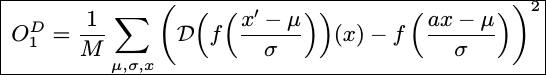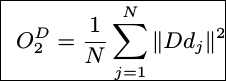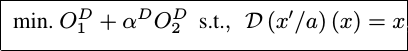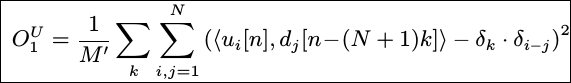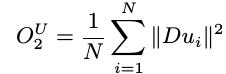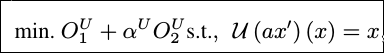I will update my understanding of this later.  For now the gist of the idea is that.  The nonlinear relation created by the biorthogonality condition is split into two linear portions in order to lower the complexity of the filter.  Part 1 satisfies the third condition of singularity preservation and the second part is to minimize power through Parsevel’s theorem.  The alpha term is to choose to prioritize either satisfying singularity or power minimization.  We choose whichever is the largest to minimize.

Source:

Freedman, Gilad, and Raanan Fattal. “Image and Video Upscaling from Local Self-Examples.” Image and Video Upscaling from Local Self-Examples(2009): n. pag. Print. http://doi.acm.org/10.1145/1559755.1559763

# Week 1: Linear Upscaling Algorithms

Week 1: 9/25-10/2/16

Definitions

• Kernel: When doing image processing using matrices the kernel is a small matrix (eg 3×3) that is multiplied with a block of pixels on the image (here 3×3) which serves as a filter.
• The multiplication mentioned above takes the 3×3 matrix of the original image multiplies each element with the corresponding m x n value in the kernel and collectively sums all values computed to a total and setting that new value as the output pixel.
• Spline: A piecewise numeric function defined by polynomials. In the case of bicubic interpolation cubic hermite splines are used where each piecewise function is a cubic polynomial of the form p(t)=(a0x^3+b0x^2+c0x+d0)*p0+…+(anx^3+bnx^2+cnx+dn)*pn

Linear VS Non linear

• Linear algorithms follow the following scheme f(a+b)=f(a)+f(b)
• This means that any input will definitely vary the output linearly
• Nonlinear algorithms such as mean and median algorithms will be able to take tare of outliers in the data allowing for a more accurate computation

Linear Upscaling Algorithms$latex \displaystyle \sum_{n=1}^\infty \frac{1}{n^2} = \frac{\pi^2}{6}.$</p> yields

Nearest Neighbor Interpolation

• Value of interpolated pixel is calculated from the nearest sample point in the input image
• Executes fast but creates a lot of artifacts
• Algorithm
• Calculate ratio of the desired increase in size of both width and height
• The ratio is orig_width/new_width
• For a 2D image use two for loops to iterate the size of the new image
• Inside for loop compute the new pixel number by doing
• pixel_x=floor(width_ratio*x_index)
• pixel_y=floor(height_ratio*y_index)
• Assign pixel to the temporary image array
• temp[x_index*new_width+y_index]=orig[pixel_y*orig_width+pixel_x]
• Return temporary image array
• This algorithm is the fastest as it doesn’t need to use any if conditionals (which is very messy in machine code due to the branches needed
• If implemented using integers instead of floating point numbers (optimization) integer division will often lead to zero
• An example of this is shrinki￼ng the image with a ratio <.5 there would be an error factor added to the ratio conditions to ensure that it wouldn’t produce a zero
• Or if we flip the ratio and edit the assignment of the pixel values it could still produce the same result

Bilinear Interpolation

• This algorithm uses instead of one nearest neighbor but four nearest neighbors to calculate the interpolated pixels
• The closer the a neighbor is the greater the influence it has on the computed pixel values
• This performs better than the single nearest neighbor algorithm however due to the linear interpolation which does not account for sharp edges or large changes in pixel data by “averaging” we create a softer image that has been blurred
• Algorithm: Given 4 points at (x1,y1), (x1, y2), (x2, y1), (x2,y2) find interpolated pixel at (x,y) where x1<x2 y1<y2 and x1<x<x2 y1<y<y2
• Compute linear interpolation in x directions
• f(x,y1)=(x2-x)/(x2-x1)*f(x1,y1)+(x-x1)/(x2-x1)*f(x2,y1)
• f(x,y2)=(x2-x)/(x2-x1)*f(x1,y2)+(x-x1)/(x2-x1)*f(x2,y2)
• Compute the linear interpolation in the y directions to complete the computation
• f(x,y)=(y2-y)/(y2-y1)*f(x,y1)+(y-y1)/(y2-y1)*f(x,y2)->Bicubic Interpolation

• Accomplished using Lagrange polynomials, cubic splines, or cubic convolution
• This is a slower algorithm than the bilinear algorithm as it needs to take into account more pixels (16 vs 4)
• Because the algorithm (like bilinear does not take into account edges) and often results in even more blurring than the bilinear interpolation algorithm
• Basic ideas:
• The whole algorithm hinges on this following equation$p(x,y)=\sum_{i=0}^{3}\sum_{j=0}^{3}a_{ij}ix^{i}y^{j}$
• This equation represents the interpolated surface
• To obtain all the values required for this equation we need to see what variables are being used
• Using a unit square on the original image we can obtain p(0,0)…p(1,1) (4 eqs)
• Which when expanded will create 16 different “a” variables indexing from 00 to 33
• These are the 16 variables we need to solve for…$p_{x}(x,y)=\sum_{i=1}^{3}\sum_{j=0}^{3}a_{ij}ix^{i-1}y^{j}$$p_{y}(x,y)=\sum_{i=0}^{3}\sum_{j=1}^{3}a_{ij}jx^{i}y^{j-1}$$p_{xy}(x,y)=\sum_{i=1}^{3}\sum_{j=1}^{3}a_{ij}ijx^{i-1}y^{j-1}$

• To solve for these 16 variables we need to solve for the x, y, and xy derivatives for all 4 points. (12 eqs) this gives us a total of 16 equations to solve for “a”
• Note that the reason why the indexing starts from 1 is because when you have “i/j”==0 the derivative constant will multiply by zero
• Now that we have all 16 equations using matrices and linear algebra we can compute all 16 “a” values.
• The above uses bicubic spline interpolation where p(x,y) is a sum of cubics
• Given the above we are now able to construct an interpolated version of the image
• An easier method would be to use a kernel which simulates the model described above• Varying the values of “a” will produce different results however the value of “-0.5=a” will produce the same result as the bicubic computation performed above
• To use this kernel to compute a bicubic interpolation for 2d images apply the transformation in the x dimension then again in the y direction$b_{-1}=p(t_x,f_(-1,-1),f_(0,-1),f_(1,-1),f_(2,-1))\newline$$b_{0}=p(t_x,f_(-1,0),f_(0,0),f_(1,0),f_(2,0))\newline$$b_{1}=p(t_x,f_(-1,1),f_(0,1),f_(1,1),f_(2,1))\newline$$b_{2}=p(t_x,f_(-1,2),f_(0,2),f_(1,2),f_(2,2))\newline$$p(x,y)=p(t_y,b_{-1},f_{0},f_{1},f_{2})$

• Indexes are as follows, t is a value from 0-1 similar to the unit circle and the b_{-1} represent the four points chosen by the bicubic interpolation->Nonlinear Algorithms

Sources:

“Bicubic Interpolation.” Wikipedia, 15 Nov. 2010. Web. 2 Oct. 2016. <https://en.wikipedia.org/wiki/Bicubic_interpolation&gt;.

“Cubic Hermite Spline.” Wikipedia, 12 Feb. 2013. Web. 2 Oct. 2016. <https://en.wikipedia.org/wiki/Cubic_Hermite_spline&gt;.

Miklós, Póth. “IMAGE INTERPOLATION TECHNIQUES.” N.p., n.d. Web. 1 Oct. 2016. <http://uni-obuda.hu/conferences/sisy2004/Poth.pdf&gt;.

“Nearest Neighbor Image Scaling.” Nearest Neighbor Image Scaling. N.p., 6 Oct. 2007. Web. 1 Oct. 2016.

“Parametric Curves.” N.p., n.d. Web. 2 Oct. 2016. <https://www.cs.cmu.edu/afs/cs/academic/class/15462-f09/www/lec/lec6.pdf&gt;.

Powell, Victor. “Image Kernels: Explained Visually.” Image Kernels: Explained Visually. N.p., n.d. Web. 30 Sept. 2016.

Singh, Gagandeep, and Prof. Gulshan Goyal. “Linear Image Upscaling :A Reveiw.” N.p., Jan. 2015. Web. 29 Sept. 2016.